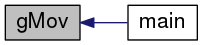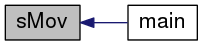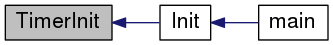heat control  r71
timer.c File Reference
`#include "timer.h"`
`#include "setup.h"`
Include dependency graph for timer.c:Go to the source code of this file.

## Functions

void TimerInit (void)
initialize timer resource
period: 32µs More...

ISR (TIMER0_COMPA_vect)
compare match interrupt service for OCR0A More...

BTN_CODE DecodeButton (void)
identify whether a (and which) button is pressed or not More...

UCHAR GetTenthSec (void)
interface to get the global tenth seconds More...

UCHAR gMov ()
return minute overflow counter More...

void sMov (UCHAR mov)
set minute overflow counter More...

## Variables

UINT volatile g_debouncer = 0

UCHAR volatile g_s100 = 0

UCHAR volatile g_sec = 0

UINT volatile g_min = 0

UCHAR volatile g_mov = 0

CTRL_MODE volatile g_ctm = CM_MEAS

ENG_TIMER volatile g_et

## Function Documentation

 BTN_CODE DecodeButton ( void )

identify whether a (and which) button is pressed or not

Parameters
 btnOff offset value, indicates the action which is active dependent on the press duration
Returns
button code which identifies the operation pattern (button(s) pressed/not pressed)

Definition at line 119 of file timer.c.

References BC_DN, BC_DNUP, BC_DNUP_L, BC_NONE, BC_UP, BTN_DN, BTN_UP, DEBOUNCED_L, DEBOUNCED_S, FALSE, g_debouncer, TRUE, UCHAR, and UINT.

Referenced by main().

Here is the caller graph for this function:UCHAR GetTenthSec ( void )

interface to get the global tenth seconds

Returns
tenth seconds

Definition at line 192 of file timer.c.

References g_s100.

Referenced by PrintPage().

Here is the caller graph for this function:UCHAR gMov ( void )

return minute overflow counter

Returns
minute overflow counter

Definition at line 200 of file timer.c.

References g_mov.

Referenced by main().

Here is the caller graph for this function:ISR ( TIMER0_COMPA_vect )

compare match interrupt service for OCR0A

Parameters
 TIMER0_COMPA_vect desired compare match interrupt vector

counter to count a tenth of a second

counter to count one second

Definition at line 72 of file timer.c.

 void sMov ( UCHAR mov )

set minute overflow counter

Parameters
 mov overflow value to set

Definition at line 208 of file timer.c.

References g_mov.

Referenced by main().

Here is the caller graph for this function:void TimerInit ( void )

initialize timer resource
period: 32µs

TCCR0B <- Timer/Counter0 Control Register B
| - | - | - | TSM  | PSR0  | CS02  | CS01  | CS00  |
clock selection:
CS02:CS00, we need a clkio division of 256

enable compare match mode

T0 match value (to get 20ms period)

enable compare match A interrupt

no PLL used

Definition at line 38 of file timer.c.

References CTC0, T0_INC20, and TCC_R0.

Referenced by Init().

Here is the caller graph for this function:## Variable Documentation

 CTRL_MODE volatile g_ctm = CM_MEAS

control mode

Definition at line 27 of file timer.c.

Referenced by DoControl(), ISR(), and main().

 UINT volatile g_debouncer = 0

debounce counter

Definition at line 7 of file timer.c.

Referenced by DecodeButton(), and ISR().

 ENG_TIMER volatile g_et

mixer engine control structure

Definition at line 31 of file timer.c.

 UINT volatile g_min = 0

number of minutes

Definition at line 19 of file timer.c.

Referenced by ISR(), and main().

 UCHAR volatile g_mov = 0

counter of overflow minutes (depends on number of measurements and the measurement interval IV_MEAS)

Definition at line 23 of file timer.c.

Referenced by gMov(), ISR(), and sMov().

 UCHAR volatile g_s100 = 0

number of tenth seconds, range is [0-9]

Definition at line 11 of file timer.c.

Referenced by GetTenthSec(), and ISR().

 UCHAR volatile g_sec = 0

number of seconds

Definition at line 15 of file timer.c.

Referenced by ISR(), and main().